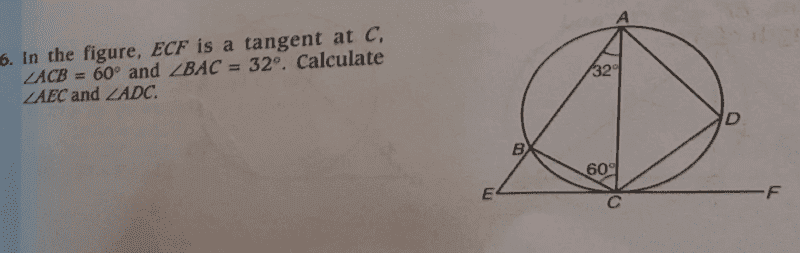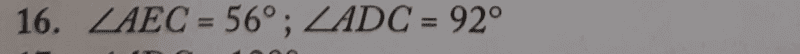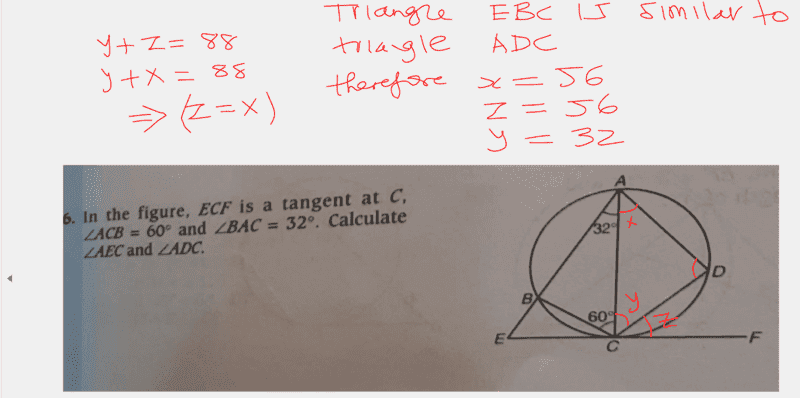# Calculate angle AEC and ADC

• chwala

#### chwala

Gold Member
Homework Statement
See attached
Relevant Equations
Deductive Geometry- Alternate segment theorem
Question;Text Solution;My reasoning;
##∠ABC= 180^0 -(32^0+60^0)##
=##88^0##

##∠ABC=∠ACF=88^0## (Alternate segment theorem).

##∠EBC=92^0## i.e angles lying on a straight line and ##∠BCE=180^0 -(88^0+60^0)=32^0## therefore;
##∠BEC=180^0 -(32^0+92^0)=56^0##

Cheers...there may be another way of looking at this...

•Lnewqban
Regarding ##∠EBC=92^0##:
Doesn't the problem figure contradicts the fact that an angle inscribed across a circle's diameter is always a right angle?

Regarding ##∠EBC=92^0##:
Doesn't the problem figure contradicts the fact that an angle inscribed across a circle's diameter is always a right angle?
...but we are not told if ##AC## is the diameter of the circle. We have only been given an indication of the tangent line, that is line ##ECF##.

•Lnewqban
Regarding ##∠EBC=92^0##:
Doesn't the problem figure contradicts the fact that an angle inscribed across a circle's diameter is always a right angle?
AC isn't a diameter, that was never mentioned

•chwala
Homework Statement:: See attached
Relevant Equations:: Deductive Geometry- Alternate segment theorem

Question;

View attachment 315298

Text Solution;

View attachment 315299

My reasoning;
##∠ABC= 180^0 -(32^0+60^0)##
=##88^0##

##∠ABC=∠ACF=88^0## (Alternate segment theorem).

##∠EBC=92^0## i.e angles lying on a straight line and ##∠BCE=180^0 -(88^0+60^0)=32^0## therefore;
##∠BEC=180^0 -(32^0+92^0)=56^0##

Cheers...there may be another way of looking at this...
You are correct that angles ##∠ACF=88^\circ## and ##∠EBC=92^\circ## .
However, your reasoning leading to determining angle ##∠BCE## is faulty, because you do not know a value for angle ##∠ACF## either.

An angle you can easily determine is ##∠ADC##. (There's a cyclic quadrilateral involved.)

You are correct that angles ##∠ACF=88^\circ## and ##∠EBC=92^\circ## .
However, your reasoning leading to determining angle ##∠BCE## is faulty, because you do not know a value for angle ##∠ACF## either.

An angle you can easily determine is ##∠ADC##. (There's a cyclic quadrilateral involved.)
@sammy but we know that ##∠ECA=∠ADC## using the alternate segment theorem. Angle ##BCE=32^0.## I do not need ##∠ACF## to determine this.

Last edited:
...Just thinking is it possible to determine all the angles in the given diagram? my thinking is as shown on the diagram below;

My reasoning being ##∠EBC## is similar to ##∠ADC## they have a common angle i.e ##92^0##.

##∠ACD=88^0 - ∠DCF=32^0##

#### Attachments

@sammy but we know that ##∠ECA=∠ADC## using the alternate segment theorem. Angle ##BCE=32^0.## I do not need ##∠ACF## to determine this.
Apologies !

I overlooked your reasoning establishing that ##∠ABC=∠ACF## .

•chwala
...Just thinking is it possible to determine all the angles in the given diagram? my thinking is as shown on the diagram below;

My reasoning being ##∠EBC## is similar to ##∠ADC## they have a common angle i.e ##92^0##.